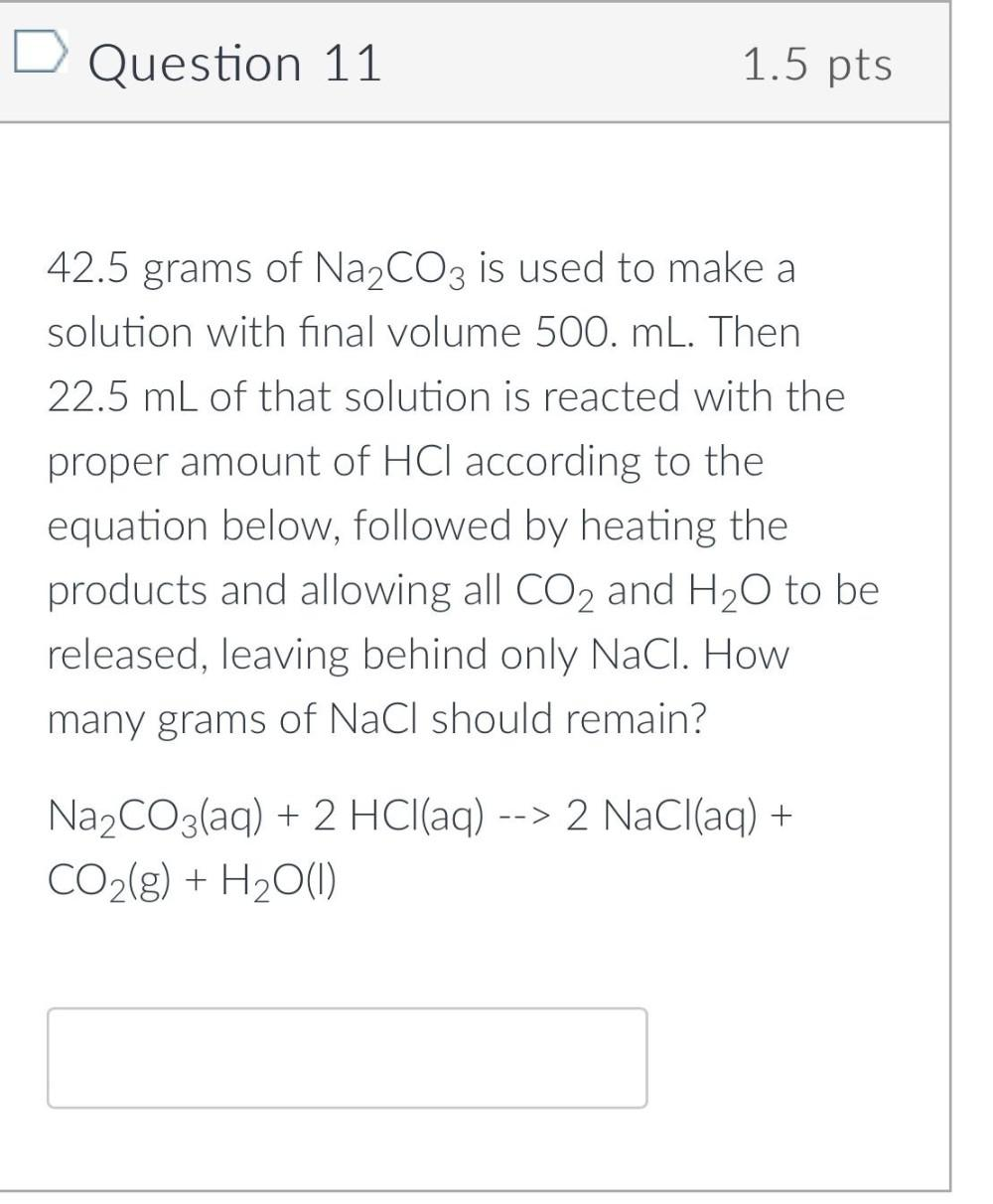Question:

# Question 11 1.5 pts 42.5 grams of Na2CO3 is used to make a solution with final volume 500. mL. Then 22.5 mL of that solution isQuestion 11 1.5 pts 42.5 grams of Na2CO3 is used to make a solution with final volume 500. mL. Then 22.5 mL of that solution is reacted with the proper amount of HCl according to the equation below, followed by heating the products and allowing all CO2 and H20 to be released, leaving behind only NaCl. How many grams of NaCl should remain? Na2CO3(aq) + 2 HCl(aq) --> 2 NaCl(aq) + CO2(g) + H2O(1)